AP State Board Syllabus AP SSC 10th Class Maths Textbook Solutions Chapter 14 Statistics Ex 14.1 Textbook Questions and Answers.

## AP State Syllabus SSC 10th Class Maths Solutions 14th Lesson Statistics Exercise 14.1

### 10th Class Maths 14th Lesson Statistics Ex 14.1 Textbook Questions and Answers

Question 1.
A survey was conducted by a group of students as a part of their environment awareness programme, in which they collected the following data regarding the number of plants in 20 houses in a locality. Find the mean number of plants per house.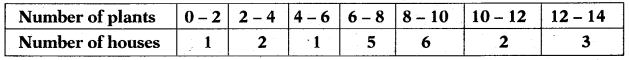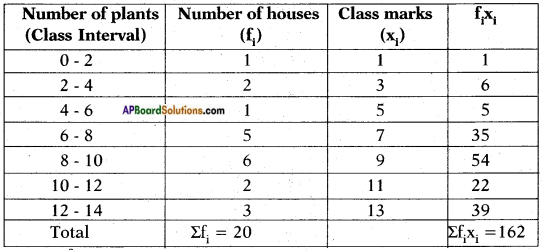Since fi and xi are of small values we use direct method.
∴ $$\overline{\mathrm{x}}=\frac{\Sigma \mathrm{f}_{\mathrm{i}} \mathrm{x}_{\mathrm{i}}}{\Sigma \mathrm{f}_{\mathrm{i}}}$$
= $$\frac{162}{20}$$
= 8.1Question 2.
Consider the following distribution of daily wages of 50 workers of a factory.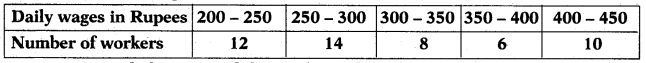Find the mean daily wages of the workers of the factory by using an appropriate method.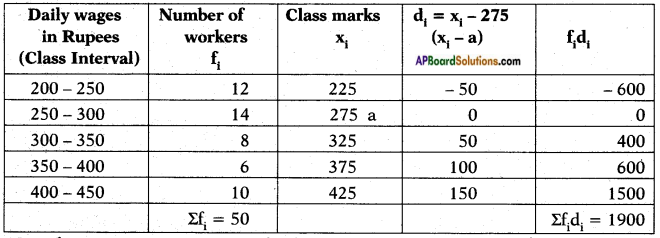Here the xi are of large numerical values.
So we use deviation method then,
$$\overline{\mathbf{x}}=\mathbf{a}+\frac{\Sigma \mathbf{f}_{\mathbf{i}} \mathbf{d}_{\mathbf{i}}}{\Sigma \mathbf{f}_{\mathbf{i}}}$$
Here the assumed mean is taken as 275.
∴ $$\overline{\mathrm{x}}=\mathrm{a}+\frac{\Sigma \mathrm{f}_{\mathrm{i}} \mathrm{d}_{\mathrm{i}}}{\Sigma \mathrm{f}_{\mathrm{i}}}$$
= 275 + $$\frac{1900}{50}$$
= 275 + 38
= 313.Question 3.
The following distribution shows the daily pocket allowance of children of a locality. The mean pocket allowance is Rs. 18. Find the missing frequency f.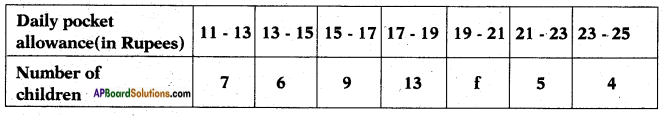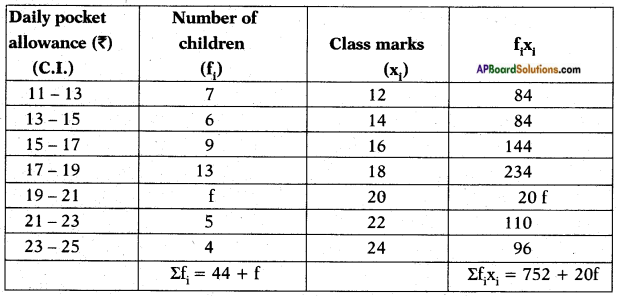$$\overline{\mathrm{x}}=\frac{\Sigma \mathrm{f}_{\mathrm{i}} \mathrm{x}_{\mathrm{i}}}{\Sigma \mathrm{f}_{\mathrm{i}}}$$
xi = 18 (given)
$$\Rightarrow 18=\frac{752+20 \mathrm{f}}{(44+\mathrm{f})}$$
18 (44 + f) = 752 + 20 f
⇒ 20f- 18f= 792-752
⇒ 2f = 40
∴ f = $$\frac{40}{20}$$ = 20.Question 4.
Thirty women were examined in a hospital by a doctor and their of heart beats per minute were recorded and summarised as shown. Find the mean heart beats per minute for these women, choosing a suitable method.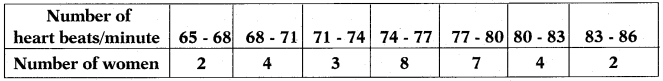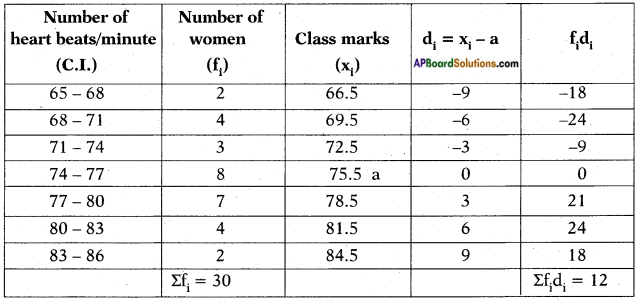$$\overline{\mathbf{x}}=\mathbf{a}+\frac{\Sigma \mathbf{f}_{\mathbf{i}} \mathbf{d}_{\mathbf{i}}}{\Sigma \mathbf{f}_{\mathbf{i}}}$$
75.5 + $$\frac{12}{30}$$
= 75.5 + 0.4
= 75.9.Question 5.
In a retail market, fruit vendors were selling oranges kept in packing baskets. These baskets contained varying number of oranges. The following was the distribution of oranges.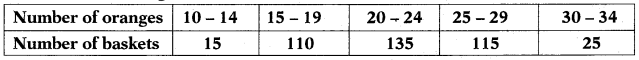Find the mean number of oranges kept in each basket. Which method of finding the mean did you choose?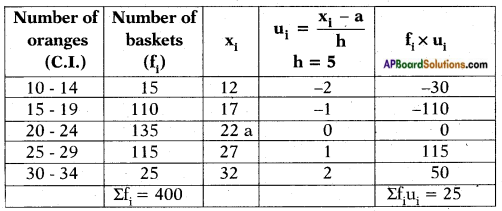Here we use step deviation method where a = 135, h = 5,a multiple of all di
$$\overline{\mathrm{x}}=\mathrm{a}+\left(\frac{\Sigma \mathrm{f}_{\mathrm{i}} \mathrm{u}_{\mathrm{i}}}{\Sigma \mathrm{f}_{\mathrm{i}}}\right) \times \mathrm{h}$$
= 22 + $$\frac{25}{400}$$ × 5
= 22 + 0.31
= 22.31Question 6.
The table below shows the daily expenditure on food of 25 households in a locality.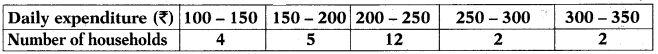Find the mean daily expenditure on food by a suitable method.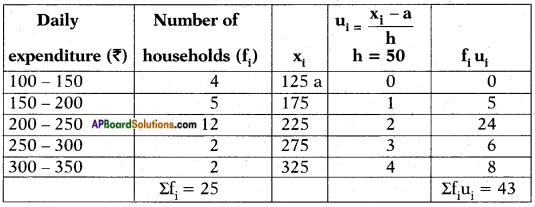Here a = 125, h = 50, ∑fiui = 43
Now
$$\overline{\mathrm{x}}=\mathrm{a}+\left(\frac{\Sigma \mathrm{f}_{\mathrm{i}} \mathrm{u}_{\mathrm{i}}}{\Sigma \mathrm{f}_{\mathrm{i}}}\right) \times \mathrm{h}$$
= 125 + $$\frac{43}{25}$$ × 50
= 125 + (43 × 2)
= 125 + 86
= 211.
NOTE: If we consider first value as “a” then we dont get negative values in ui, fiui columns. Then it becomes easy for calculation.Question 7.
To find out the concentration of SO2 in the air (in parts per million, i.e., ppm), the data was collected for 30 localities in a certain city and is presented below: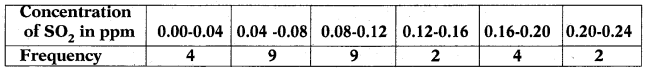Find the mean concentration of SO2 in the air.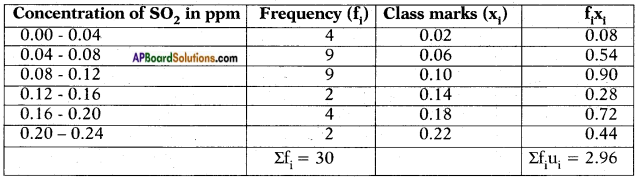∴ $$\overline{\mathrm{x}}=\frac{\Sigma \mathrm{f}_{\mathrm{i}} \mathrm{x}_{\mathrm{i}}}{\Sigma \mathrm{f}_{\mathrm{i}}}$$
= $$\frac{2.96}{30}$$
= 0.00986666…….
≃ 0.099Question 8.
A class teacher has the following attendance record of 40 students of a class for the whole term. Find the mean number of days a student was present out of 56 days in the term.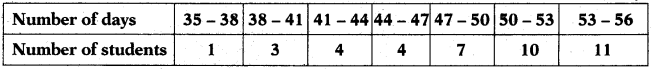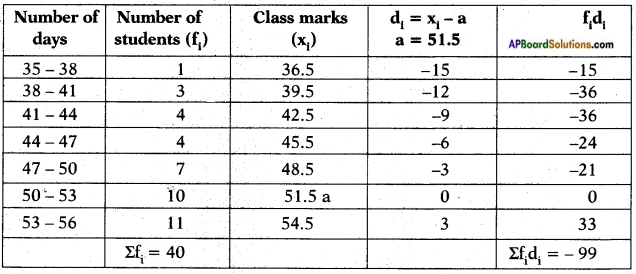Here, a = 51.5
∴ $$\overline{\mathbf{x}}=\mathbf{a}+\frac{\Sigma \mathbf{f}_{\mathbf{i}} \mathbf{d}_{\mathbf{i}}}{\Sigma \mathbf{f}_{\mathbf{i}}}$$
= 51.5 – $$\frac{99}{40}$$
= 51.5 – 2.475
= 49.025
≃ 49 daysQuestion 9.
The following table gives the literacy rate (in percentage) of 35 cities. Find the mean literacy rate.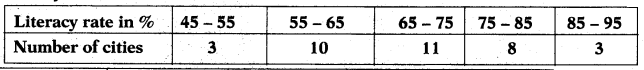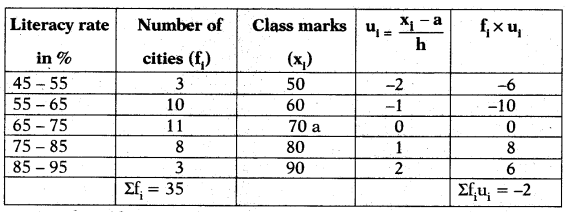∴ $$\overline{\mathbf{x}}=\mathbf{a}+\frac{\Sigma \mathbf{f}_{\mathbf{i}} \mathbf{u}_{\mathbf{i}}}{\Sigma \mathbf{f}_{\mathbf{i}}} \times \mathbf{h}$$
$$\Rightarrow \bar{x}=70-\frac{2}{35} \times 10$$
= 70 – $$\frac{2}{35}$$ × 10
= 70 – $$\frac{20}{35}$$